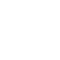# Charles’s Law, Formula, and Key Points to Know.

Charles’s law states that the volume of a given mass of gas is directly proportional to its absolute temperature when pressure is kept constant. This means that if the temperature of a gas is increased, its volume will increase proportionally. Conversely, if the temperature of a gas is decreased, its volume will decrease proportionally.

# Charles’s Law:

Charles’s law states that the volume of a given mass of gas is directly proportional to its absolute temperature when pressure is kept constant. This means that if the temperature of a gas is increased, its volume will increase proportionally. Conversely, if the temperature of a gas is decreased, its volume will decrease proportionally. It complements other gas laws like Bayle’s law and Avogadro’s law.

The absolute temperature is measured in Kelvins. The Kelvin scale is a temperature scale based on the absolute zero of temperature, which is the temperature at which all molecular motion ceases.

## Formula for Charles’s law:

The mathematical formula for Charles’s law is:

V1/T1 = V2/T2

Where,

• V1 is the initial volume of the gas
• T1 is the initial temperature of the gas in Kelvin
• V2 is the final volume of the gas
• T2 is the final temperature of the gas in Kelvins

For example, if a gas has an initial volume of 1 liter and an initial temperature of 273 K, and its temperature is then increased to 373 K, its final volume will be 2 liters.

In this case, we have:

• V1 = 1 litre
• T1 = 273 K
• T2 = 373 K

Substituting these values into the formula, we get:

V1 / T1 = V2 / T2

1 / 273 = V2 / 373

V2 = (1 * 373) / 273

V2 = 2 litres

Charles’s law is a fundamental law of physics that is used in many applications, such as weather forecasting, air conditioning, and the design of balloons and airships.

## Key Points in Understanding the Law:

1.Charles’s Law states that the volume of a gas is directly proportional to its absolute temperature when the pressure and the amount of gas are held constant.

2.The Kelvin temperature scale is used in conjunction with Charles’s Law because it is an absolute temperature scale where 0 K (absolute zero) represents the point at which all molecular motion theoretically stops.

3.Some practical applications of Charles’s Law include hot air balloons, airbags in vehicles, and laboratory experiments.

4.Charles’s Law is one of the three gas laws that are commonly combined to form the ideal gas law.

5.Understanding Charles’s Law is crucial in industries where gases are stored, transported, or used.

6.The direct proportionality of volume and temperature means that if the volume of a gas doubles, its temperature will also double. Conversely, if the temperature of a gas is halved, its volume will also be halved.

7.The Kelvin temperature scale is used because it is based on the kinetic theory of gases, which states that the temperature of a gas is a measure of the average kinetic energy of its molecules.

8.Hot air balloons work because the air inside the balloon is less dense than the surrounding air. As the air inside the balloon is heated, its volume increases and its density decreases. This causes the balloon to rise, as it is less dense than the surrounding air.

9.Airbags in vehicles work by using the same principle as hot air balloons. When a car’s airbag deploys, a chemical reaction is triggered that generates gas. The gas’s volume increases and its density decreases, causing the airbag to inflate rapidly.

10.Laboratory experiments involving Charles’s Law typically involve a gas trapped in a flexible container. As the gas is heated or cooled, its volume changes in accordance with the law.

11.In industries where gases are stored, transported, or used, it is important to understand how the properties of gases will change under different temperature conditions. This knowledge can be used to ensure the safety and operational efficiency of these processes.

Remember that Charles’s Law is most accurate when dealing with ideal gases under certain conditions, and deviations can occur in real-world scenarios, especially at high pressures and low temperatures

## Conclusion:

Remember that Charles’s Law is most accurate when dealing with ideal gases under certain conditions, and deviations can occur in real-world scenarios, especially at high pressures and low temperatures.

•August 26, 2023 at 4:26 pm
Dillip kumar Pramanik

Very clearly explained👌👌